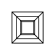# 带你十分钟快速入门画图神器 MatplotlibMatplotlib 是 Python 的一个绘图库。它包含了大量的工具，你可以使用这些工具创建各种图形，包括简单的散点图，正弦曲线，甚至是三维图形。

## 目录

1. 画一个简单的图形

2. 在一张图纸里绘制多个图形

3. 更多设置

3.1 设置 figure

3.2 设置标题

3.3 设置坐标轴

3.4 设置 label 和 legend

3.5 添加注释

4. 使用子图

5. 常见的图形

5.1 散点图

5.2 柱状图

6. 中文乱码解决

# 导入相关模块import matplotlib.pyplot as pltimport numpy as np

## 画一个简单的图形

x = np.linspace(0, 2 * np.pi, 50)y = np.sin(x)

plt.plot(x, y)plt.show()

## 在一张图纸里绘制多个图形

plt.plot(x, y)plt.plot(x, y * 2)plt.show()

plt.plot(x, y, 'y*-')plt.plot(x, y * 2, 'm--')plt.show()

.

o

## 更多设置

Matplotlib 支持各种灵活的设置，这里我们列举一些常见的内容。

### 设置 figure

plt.figure(figsize=(6, 3))plt.plot(x, y)plt.plot(x, y * 2)plt.show()

### 设置标题

plt.plot(x, y)plt.plot(x, y * 2)plt.title("sin(x) & 2sin(x)")plt.show()

### 设置坐标轴

plt.plot(x, y)plt.plot(x, y * 2)plt.xlim((0, np.pi + 1))plt.ylim((-3, 3))plt.xlabel('X')plt.ylabel('Y')plt.show()

plt.plot(x, y)plt.plot(x, y * 2)plt.xticks((0, np.pi * 0.5, np.pi, np.pi * 1.5, np.pi * 2))plt.show()

### 设置 label 和 legend

plt.plot(x, y, label="sin(x)")plt.plot(x, y * 2, label="2sin(x)")# plt.legend()plt.legend(loc='best')plt.show()

### 添加注释

plt.plot(x, y)x0 = np.piy0 = 0# 画出标注点plt.scatter(x0, y0, s=50)plt.annotate('sin(np.pi)=%s' % y0, xy=(np.pi, 0), xycoords='data', xytext=(+30, -30),             textcoords='offset points', fontsize=16,             arrowprops=dict(arrowstyle='->', connectionstyle="arc3,rad=.2"))plt.text(0.5, -0.25, "sin(np.pi) = 0", fontdict={'size': 16, 'color': 'r'})plt.show()

• 'sin(np.pi)=%s' % y0 代表标注的内容，可以通过字符串 %s 将 y0 的值传入字符串；

• 参数 xycoords='data' 是说基于数据的值来选位置;

• xytext=(+30, -30)  textcoords='offset points' 表示对于标注位置的描述 和 xy 偏差值，即标注位置是 xy 位置向右移动 30，向下移动30；

• arrowprops 是对图中箭头类型和箭头弧度的设置，需要用 dict 形式传入。

## 使用子图

ax1 = plt.subplot(2, 2, 1) # （行，列，活跃区）plt.plot(x, np.sin(x), 'r')ax2 = plt.subplot(2, 2, 2, sharey=ax1) # 与 ax1 共享y轴plt.plot(x, 2 * np.sin(x), 'g')ax3 = plt.subplot(2, 2, 3)plt.plot(x, np.cos(x), 'b')ax4 = plt.subplot(2, 2, 4, sharey=ax3) # 与 ax3 共享y轴plt.plot(x, 2 * np.cos(x), 'y')plt.show()

ax1 = plt.subplot(2, 1, 1) # （行，列，活跃区）plt.plot(x, np.sin(x), 'r')ax2 = plt.subplot(2, 3, 4)plt.plot(x, 2 * np.sin(x), 'g')ax3 = plt.subplot(2, 3, 5, sharey=ax2)plt.plot(x, np.cos(x), 'b')ax4 = plt.subplot(2, 3, 6, sharey=ax2)plt.plot(x, 2 * np.cos(x), 'y')plt.show()

## 常见的图形

### 散点图

k = 500x = np.random.rand(k) y = np.random.rand(k)size = np.random.rand(k) * 50 # 生成每个点的大小colour = np.arctan2(x, y) # 生成每个点的颜色大小plt.scatter(x, y, s=size, c=colour)plt.colorbar() # 添加颜色栏plt.show()

### 柱状图

k = 10x = np.arange(k)y = np.random.rand(k)plt.bar(x, y) # 画出 x 和 y 的柱状图# 增加数值for x, y in zip(x, y):    plt.text(x, y , '%.2f' % y, ha='center', va='bottom')plt.show()

## 中文乱码解决

Matplotlib 有个让人恼火的问题是，默认情况下，Matplotlib 中文会乱码。

x = ['北京', '上海', '深圳', '广州']y = [60000, 58000, 50000, 52000]plt.plot(x, y)plt.show()

plt.rcParams['font.sans-serif']=['SimHei'] #用来正常显示中文标签plt.rcParams['axes.unicode_minus']=False #用来正常显示负号plt.plot(x, y)plt.show()「若你有原创文章想与大家分享，欢迎投稿。」07-021万+
07-14239
06-23362
12-19105
04-231561
04-301389
03-2970
04-12725
04-0612
02-25294
05-231121
12-241万+
10-25329
04-271万+
01-251347
02-25310
01-048万+
05-12895
08-107490

### “相关推荐”对你有帮助么？

•非常没帮助
•没帮助
•一般
•有帮助
•非常有帮助被折叠的  条评论 为什么被折叠?到【灌水乐园】发言点击重新获取扫码支付1.余额是钱包充值的虚拟货币，按照1:1的比例进行支付金额的抵扣。
2.余额无法直接购买下载，可以购买VIP、C币套餐、付费专栏及课程。余额充值